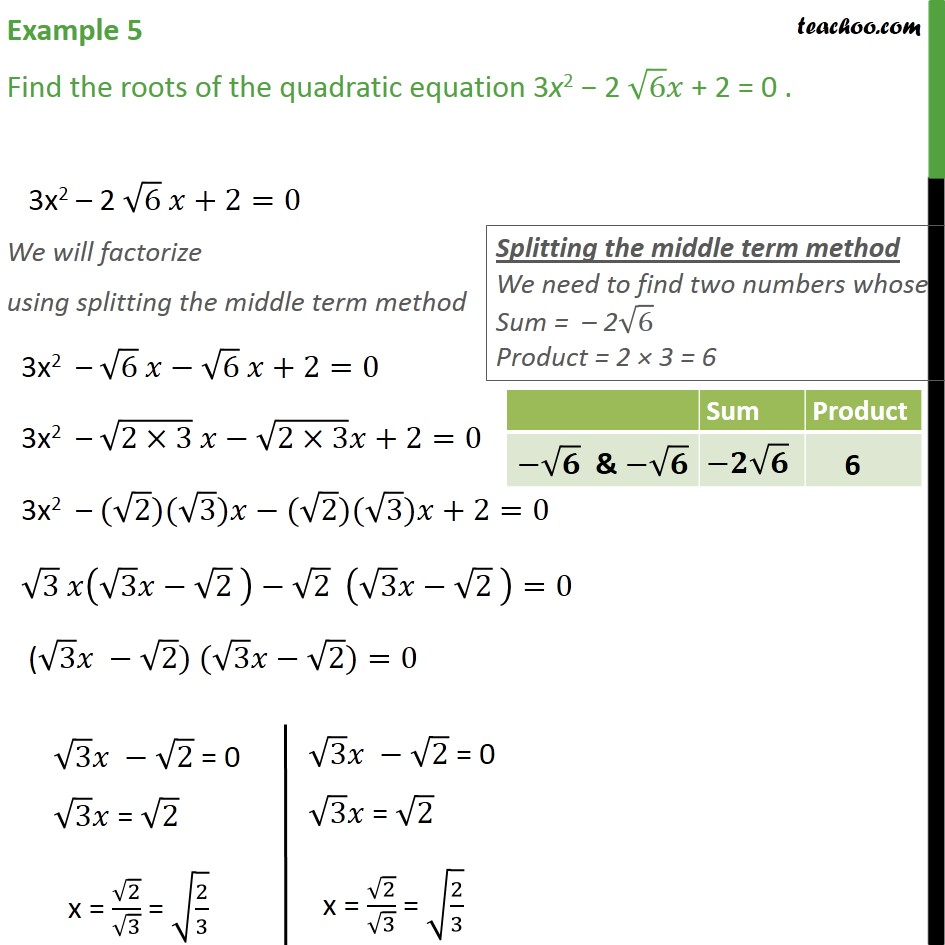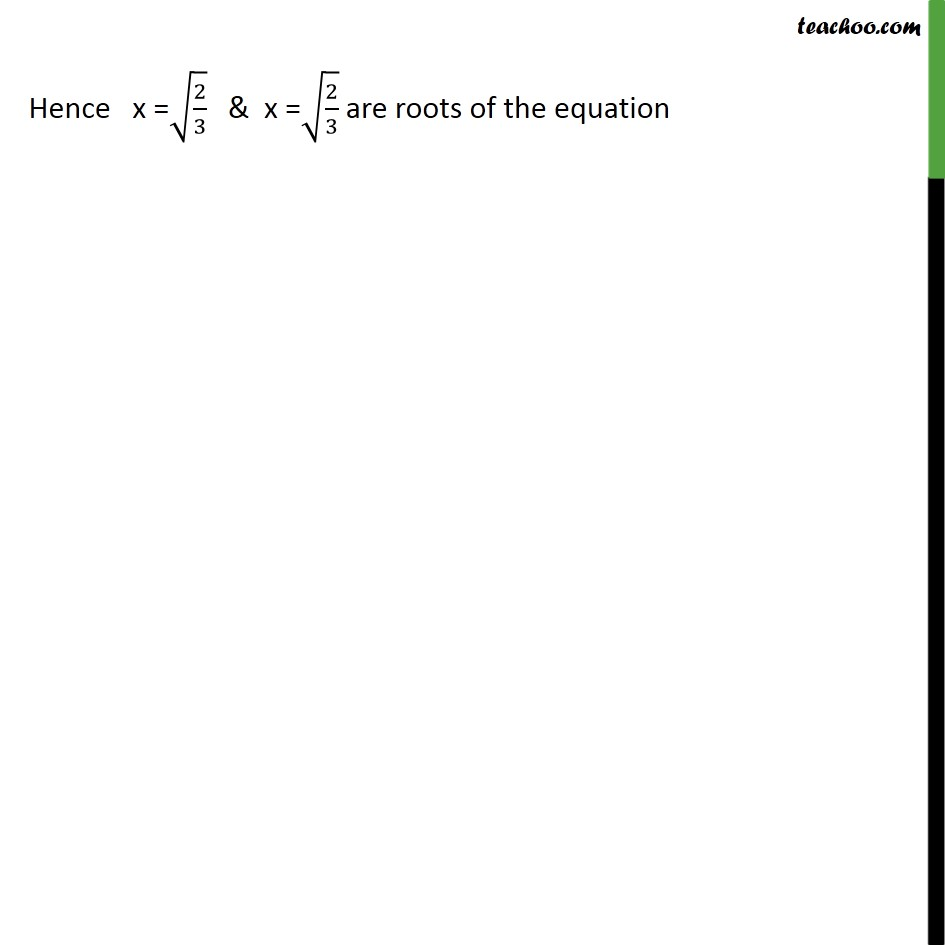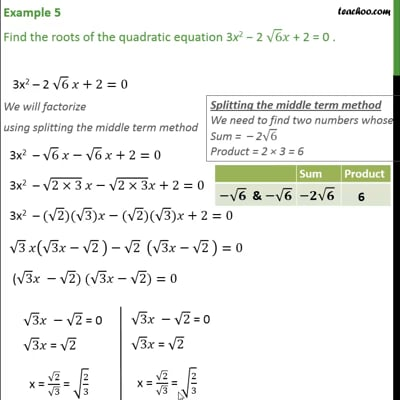Chapter 4 Class 10 Quadratic Equations

Class 10
Important Questions for Exam - Class 10This video is only available for Teachoo black users

Introducing your new favourite teacher - Teachoo Black, at only ₹83 per month

### Transcript

Example 5 Find the roots of the quadratic equation 3x2 − 2 √6 𝑥 + 2 = 0 . 3x2 – 2 √6 𝑥+2=0 We will factorize using splitting the middle term method 3x2 – √6 𝑥−√6 𝑥+2=0 3x2 – √(2×3) 𝑥−√(2×3) 𝑥+2=0 3x2 – (√2)(√3)𝑥−(√2)(√3)𝑥+2=0 √3 𝑥(√3 𝑥−√2 )−√2 (√3 𝑥−√2 )=0 (√3 𝑥 −√2) (√3 𝑥−√2)=0 Hence x =√(2/3) & x =√(2/3) are roots of the equation